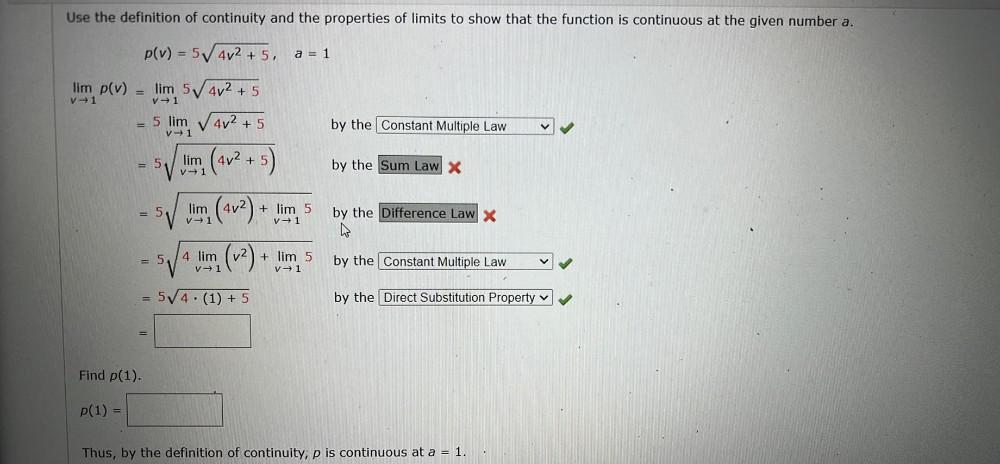Question:

# Use the definition of continuity and the properties of limits to show that the function is continuous at the given number a. p(vUse the definition of continuity and the properties of limits to show that the function is continuous at the given number a. p(v) = 5402 + 5, = a = 1 lim p(v) V +1 V1 lim 5 4v2 + 5 5 lim V 4v2 + 5 by the Constant Multiple Law V1 = 5 lim (4v2 + 5 by the Sum Law X V1 = 5 lim V1 + lim 5 V1 by the Difference Law X (4v2) + (12) + 5 4 lim V1 + lim 5 V1 by the Constant Multiple Law 5/4 (1) + 5 + by the Direct Substitution Property v Find p(1) P(1) = Thus, by the definition of continuity, p is continuous at a = 1.